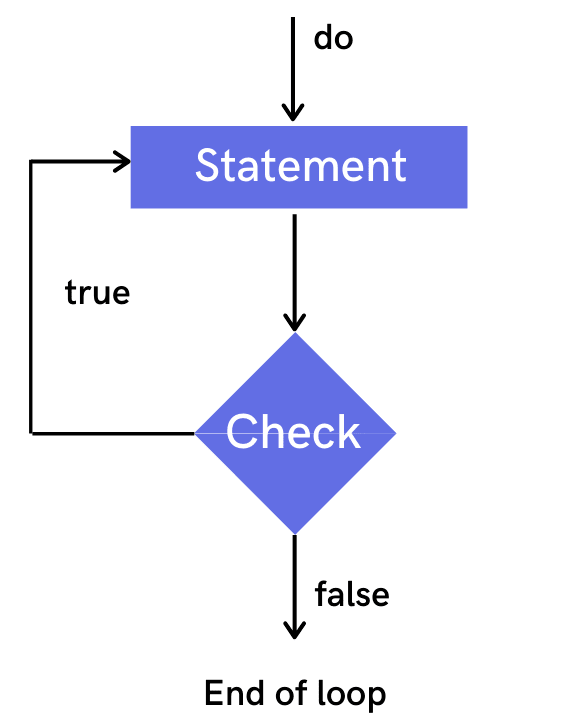# JAVA DO-WHILE LOOP

Do While Loop in Java functions the same as that of while loop but there is a difference between them in terms of condition evaluation. In the while loop, we evaluated the condition before the execution of the loop's body while in do-while the condition is evaluated after the execution of the loop's body.

[hide]
• Do While Loop in Java
• Example :A Simple Do While Program
• Example :Factorial using Do While loop

## Do While loop in Java

Loops in Java Programming Language is a way to efficiently write a code that will iterate over a part of block multiple times if the condition is true.

If we are certain that we have to execute the code block once and the number of iteration is not fixed, then it is always recommended to use do-while loop. It is executed once since the condition is always checked after the completion of loop's body.

### Java Do While loop Syntax

`do {// Code block inside do while loop } while (condition check);`

### Working :

In the above syntax first code inside the do-while loop gets executed and then the conditional block gets evaluated, the condition returns a boolean value i.e true or false. If the condition is true then control transfers to the "do" else the loop gets terminated. Lets see the working of do while loop with the help of flow diagram below.

### Flow DiagramWhile working with a do-while loop, the increment, and decrement of loop variables happen inside the while loop so that values get changed on each iteration and we could get false at some point to terminate a loop.

## A Simple Do While Program

Here we will print the values from 1 to n using do while loop. This is one of the basic program for the understanding perspective of the flow.``````// Java program to demonstrate do while loop example
import java.util.*;
public class DoWhileExample{
public static void main(String args[]){
int i = 1;
Scanner sc= new Scanner(System.in);
System.out.print("Enter any number : ");
int num = sc.nextInt();

do{
System.out.print(i+" ");
i++;
} while(i<=num);
}
}``````
Enter any number : 12

#### OUTPUT :

`1 2 3 4 5 6 7 8 9 10 11 12`

Since this is the basic program, hence we are not going dry run it. kindly try it by yourself. If facing any difficulty kindly watch our Java Control Statements Loops modules in which we have shown how to dry run the given programs on paper with the help of tabular representation.

## Factorial using Do While loop

If you don't know what is Factorial kindly go through Java For loop Factorial Example. We have already solved the factorial problem using a while loop in the previous tutorial. If not seen yet then kindly go through Java While Loop Examples. Here we are going to solve the same problem with the help of a do-while loop so that you can get the basic understanding of how while and do-while loop works.``````// Java program to calculate factorial of any number using do while
import java.util.*;
public class FactorialExample{
public static void main(String args[]){
int fact = 1;
Scanner sc= new Scanner(System.in);
System.out.print("Enter any number : ");
int num = sc.nextInt();

do {
fact = fact * num;
num--;
}  while(num > 0);
System.out.println( "Factorial is : " + fact);
}
}``````
Enter any number : 5

#### OUTPUT :

`Factorial is : 120`

### Dry Run

 Initialization Do while loop body Condition check Print Values fact = 1; num= 5; fact = 1 * 5 = 5 ; num = 4 4 > 0 // true fact = 5; num= 4; fact = 5 * 4 = 20 ; num = 3 3 > 0 // true fact = 20; num= 3; fact = 20 * 3 = 60 ; num = 2 2 > 0 // true fact = 60; num= 2; fact = 60 * 2 = 120 ; num = 1 1 > 0 // true fact = 120; num= 1; fact = 120 * 1 = 120 ; num = 0 0 > 0 // false Factorial is :120

In this example, we have taken the input as 5. Kindly give different values and then dry run your program with pen and paper to understand the flow better.